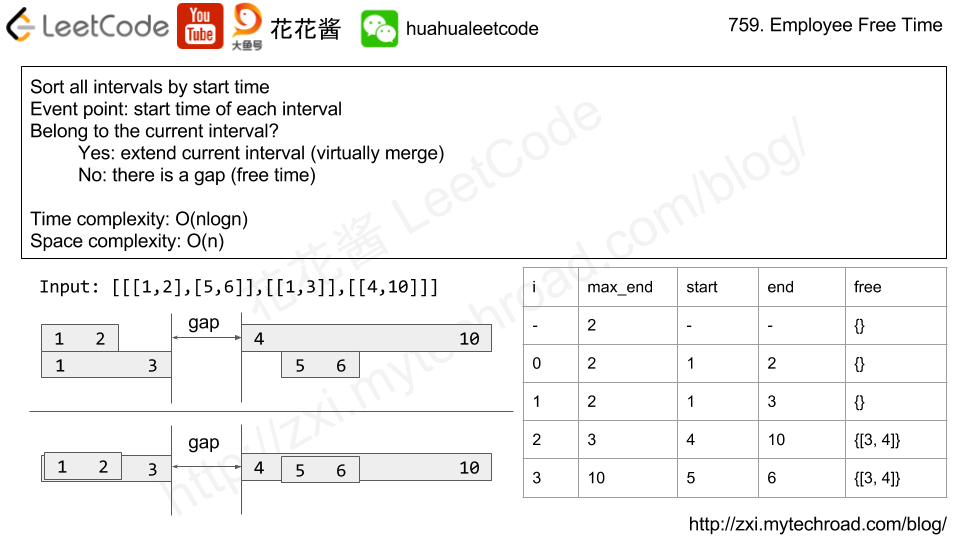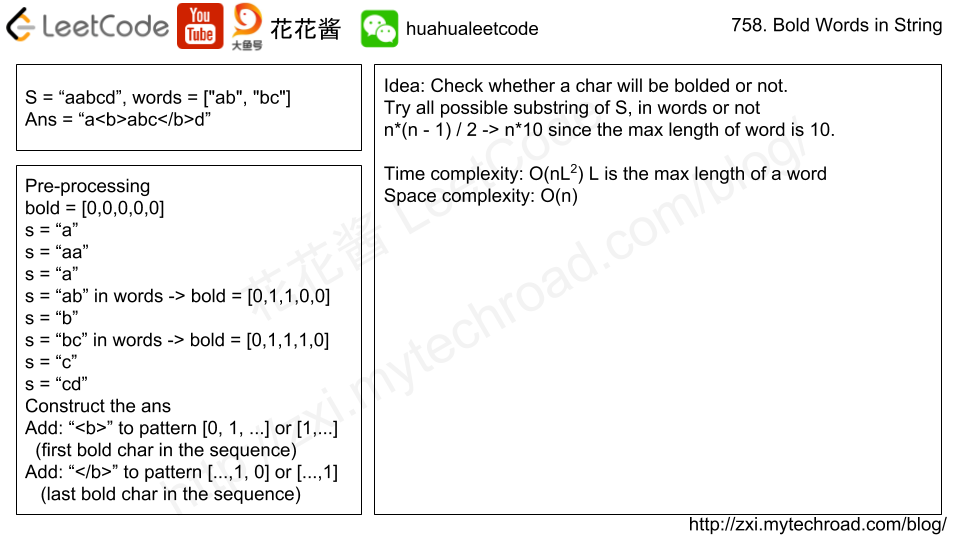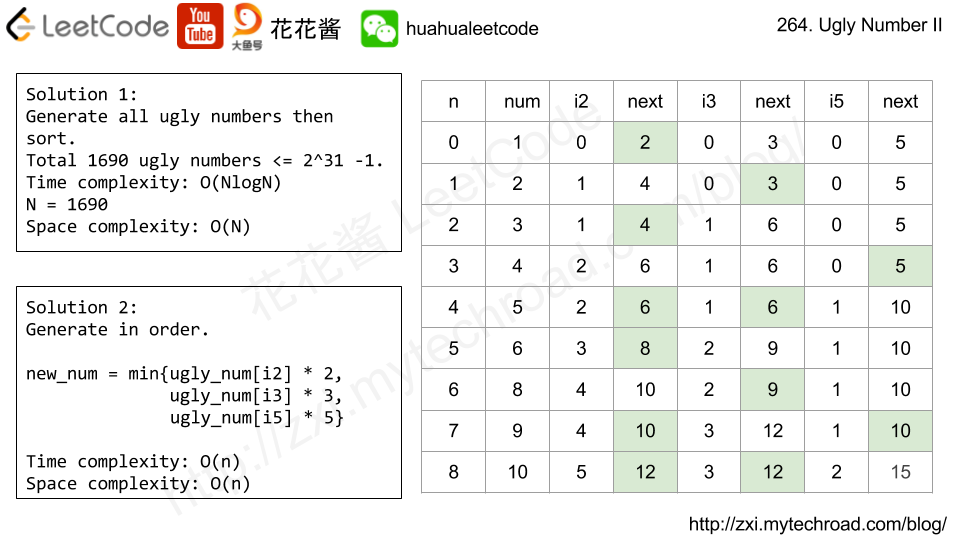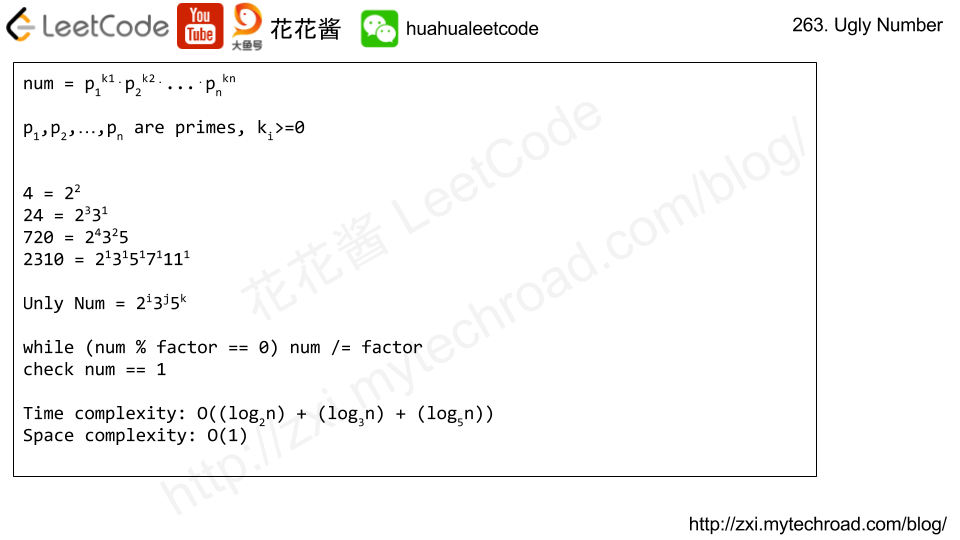Problem:

We are given a list schedule of employees, which represents the working time for each employee.

Each employee has a list of non-overlapping Intervals, and these intervals are in sorted order.

Return the list of finite intervals representing common, positive-length free time for all employees, also in sorted order.

Example 1:

Example 2:

(Even though we are representing Intervals in the form [x, y], the objects inside are Intervals, not lists or arrays. For example, schedule.start = 1, schedule.end = 2, and schedule is not defined.)

Also, we wouldn’t include intervals like [5, 5] in our answer, as they have zero length.

Note:

1. schedule and schedule[i] are lists with lengths in range [1, 50].
2. 0 <= schedule[i].start < schedule[i].end <= 10^8.

Idea:

Merge Intervals (virtually)Solution:

C++

Time complexity: O(nlogn)

Space complexity: O(n)

n is the total number of intervals, n <= 2500

Related Problems:

Problem:

Given a set of keywords words and a string S, make all appearances of all keywords in S bold. Any letters between <b> and </b> tags become bold.

The returned string should use the least number of tags possible, and of course the tags should form a valid combination.

For example, given that words = ["ab", "bc"] and S = "aabcd", we should return "a<b>abc</b>d". Note that returning "a<b>a<b>b</b>c</b>d" would use more tags, so it is incorrect.

Note:

1. words has length in range [0, 50].
2. words[i] has length in range [1, 10].
3. S has length in range [0, 500].
4. All characters in words[i] and S are lowercase letters.Solution:

C++

Time complexity: O(nL^2)

Space complexity: O(n + d)

d: size of dictionary

L: max length of the word which is 10.

Given two lists Aand B, and B is an anagram of AB is an anagram of A means B is made by randomizing the order of the elements in A.

We want to find an index mapping P, from A to B. A mapping P[i] = j means the ith element in A appears in B at index j.

These lists A and B may contain duplicates. If there are multiple answers, output any of them.

For example, given

We should return

as P = 1 because the 0th element of A appears at B, and P = 4 because the 1st element of Aappears at B, and so on.

Solution:

C++

Time complexity: O(nlogn)

Space complexity: O(n)

C++ / No duplication

Problem:

Write a program to find the n-th ugly number.

Ugly numbers are positive numbers whose prime factors only include 2, 3, 5. For example, 1, 2, 3, 4, 5, 6, 8, 9, 10, 12 is the sequence of the first 10 ugly numbers.

Note that 1 is typically treated as an ugly number, and n does not exceed 1690.Solution:

C++ / O(n)

C++ /query * O(NlogN)

C++ / static variables O(NlogN) + query*O(1)

C++ / table O(1)
leetcode_264_table.cc

# Related Problems:

Problem:

Write a program to check whether a given number is an ugly number.

Ugly numbers are positive numbers whose prime factors only include 2, 3, 5. For example, 6, 8 are ugly while 14 is not ugly since it includes another prime factor 7.

Note that 1 is typically treated as an ugly number.

Idea:

MathSolution:

C++

Related Problems:

Mission News Theme by Compete Themes.